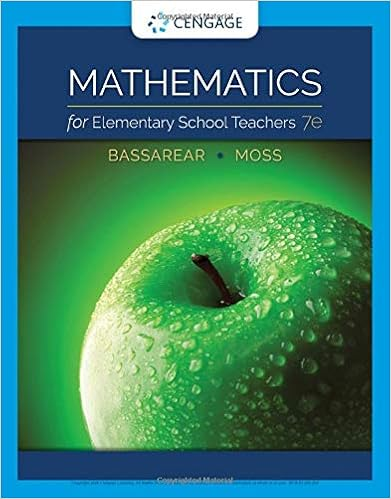# N diverges so by the comparison test the series ln n

• Homework Help
• 4
• 87% (15) 13 out of 15 people found this document helpful

This preview shows page 1 - 3 out of 4 pages.

##### We have textbook solutions for you!
The document you are viewing contains questions related to this textbook.The document you are viewing contains questions related to this textbook.
Chapter 3 / Exercise 23
Mathematics for Elementary School Teachers
BassarearExpert Verified
ndiverges, soby the Comparison Test, the seriesln(n)ndiverges.Write your answer asa fully simplified fraction. For6. For alln>2,ln(n)n2>n2, and the seriesn2converges,so by the Comparison Test, the seriesln(n)n2converges.an+1
IIICC
3.(1 pt)Consider the seriesn=11n(n+3)Determine whether the series converges, and if it converges,determine its value.Converges (y/n):Value if convergent (blank otherwise):Correct Answers:
4.(1 pt) Match each of the following with the correct state-ment.C stands for Convergent, D stands for Divergent.1.8n2.n=112+4n63.n=22n8-94.n=12n(n+3)5.n=18+7n8+2nn=1ln(n)
DCCC
5.(1 pt) Use the ratio test to determine whethern!converges or diverges.
(a) Find the ratio of successive terms.Write your answer asa fully simplified fraction. For
an+1
##### We have textbook solutions for you!
The document you are viewing contains questions related to this textbook.The document you are viewing contains questions related to this textbook.
Chapter 3 / Exercise 23
Mathematics for Elementary School Teachers
BassarearExpert Verified
(b) Evaluate the limit in the previous part. Enterasinfinityand-as-infinity.If the limit does not exist, enterDNE.limnan+1an=(c) By the ratio test, does the series converge, diverge, or isthe test inconclusive??
6.(1 pt) Use the ratio test to determine whether2nconverges or diverges.(a) Find the ratio of successive terms.Write your answer asa fully simplified fraction. For
(b) Evaluate the limit in the previous part. Enterasinfinityand-as-infinity.If the limit does not exist, enterDNE.limn1an=(c) By the ratio test, does the series converge, diverge, or isthe test inconclusive??
•••